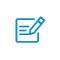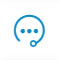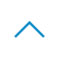# 25 个有用的Python代码段

Python 是一种通用的高级编程语言。用它可以做许多事，比如开发桌面 GUI 应用程序、网站和 Web 应用程序等。1. 在两个变量之间交换值

a = 5
b = 10
a,b = b,a
print(a) # 10
print(b) # 5

2. 检查给定的数字是否为偶数

def is_even(num):
return num % 2 == 0

is_even(10) # True

3. 将多行字符串拆分为行列表

def split_lines(s):
return s.split('\n')
split_lines('50\n python\n snippets') # ['50', ' python', ' snippets']

4. 查找对象使用的内存

import sys
print(sys.getsizeof(5)) # 28
print(sys.getsizeof("Python")) # 55

5. 反转字符串

Python 字符串库不像其他 Python 容器（如 list) 那样支持内置的 reverse()。反转字符串有很多方法，其中最简单的方法是使用切片运算符（slicing operator）。

language = "python"
reversed_language = language[::-1]
print(reversed_language) # nohtyp

6. 打印字符串 n 次

def repeat(string, n):
return (string * n)

repeat('python', 3) # pythonpythonpython

7. 检查字符串是否为回文

def palindrome(string):
return string == string[::-1]

palindrome('python') # False

8. 将字符串列表合并为单个字符串

strings = ['50', 'python', 'snippets']
print(','.join(strings)) # 50,python,snippets

9. 查找列表的第一个元素

return list

print(head([1, 2, 3, 4, 5])) # 1

10. 查找存在于两个列表中任一列表存在的元素

def union(a,b):
return list(set(a + b))
union([1, 2, 3, 4, 5], [6, 2, 8, 1, 4]) # [1,2,3,4,5,6,8]

11. 查找给定列表中的所有唯一元素

def unique_elements(numbers):
return list(set(numbers))

unique_elements([1, 2, 3, 2, 4]) # [1, 2, 3, 4]

12. 求一组数字的平均值

def average(*args):
return sum(args, 0.0) / len(args)

average(5, 8, 2) # 5.0

13. 检查列表是否包含所有唯一值

def unique(list):
if len(list)==len(set(list)):
print("All elements are unique")
else:
print("List has duplicates")

unique([1,2,3,4,5]) # All elements are unique

14. 跟踪列表中元素的频率

Python 计数器跟踪容器中每个元素的频率。 Counter() 返回一个以元素为键、以其出现频率为值的字典。

from collections import Counter
list = [1, 2, 3, 2, 4, 3, 2, 3]
count = Counter(list)
print(count) # {2: 3, 3: 3, 1: 1, 4: 1}

15. 查找列表中最常用的元素

def most_frequent(list):
return max(set(list), key = list.count)

numbers = [1, 2, 3, 2, 4, 3, 1, 3]
most_frequent(numbers) # 3

16. 将角度转换为弧度

import math
return (deg * math.pi) / 180.0

17. 计算执行一段代码所需的时间

import time
start_time = time.time()
a,b = 5,10
c = a+b
end_time = time.time()
time_taken = (end_time- start_time)*(10**6)
print("Time taken in micro_seconds:", time_taken) # Time taken in micro_seconds: 39.577484130859375

18. 查找数字列表的最大公约数

from functools import reduce
import math
def gcd(numbers):
return reduce(math.gcd, numbers)
gcd([24,108,90]) # 6

19. 查找字符串中的唯一字符

string = "abcbcabdb"
unique = set(string)
new_string = ''.join(unique)
print(new_string) # abcd

20. 使用 lambda 函数

Lambda 是一个匿名函数，它只能保存一个表达式。

x = lambda a, b, c : a + b + c
print(x(5, 10, 20)) # 35

21. 使用映射函数

def multiply(n):
return n * n

list = (1, 2, 3)
result = map(multiply, list)
print(list(result)) # {1, 4, 9}

22. 使用筛选函数

arr = [1, 2, 3, 4, 5]
arr = list(filter(lambda x : x%2 == 0, arr))
print (arr) # [2, 4]

23. 使用列表解析

numbers = [1, 2, 3]
squares = [number**2 for number in numbers]
print(squares) # [1, 4, 9]

24. 使用切片运算符

def rotate(arr, d):
return arr[d:] + arr[:d]

if __name__ == '__main__':
arr = [1, 2, 3, 4, 5]
arr = rotate(arr, 2)
print (arr) # [3, 4, 5, 1, 2]

25. 使用函数链式调用

return a + b
def subtract(a, b):
return a - b
a, b = 5, 10
print((subtract if a > b else add)(a, b)) # 15

-->扫码入群咨询反馈返回顶部